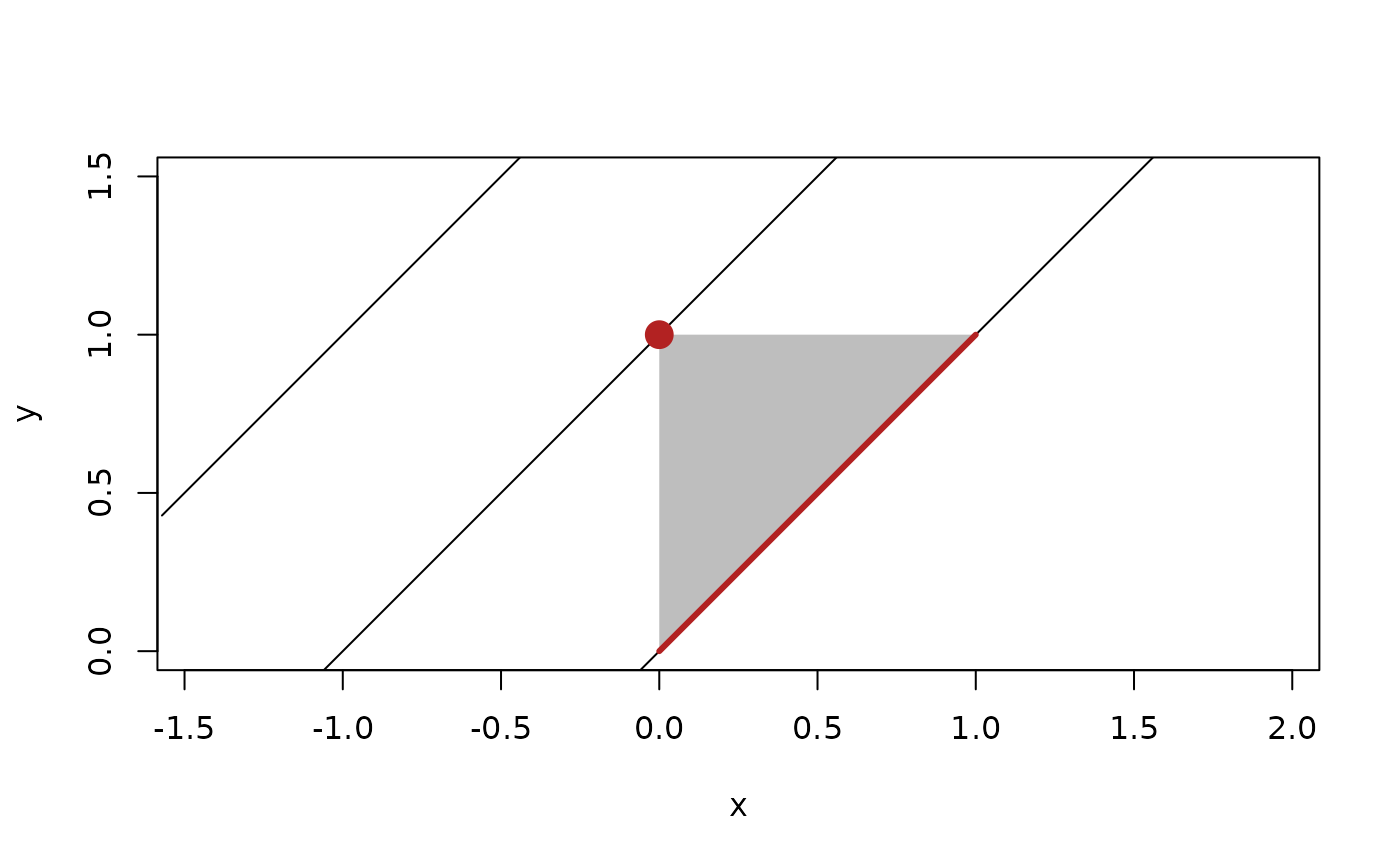An intersection between two geometries is defined as the geometry that is contained in both geometries. In other words all points laying on the intersection is also part of the two incoming geometries. It follows that the result of calculating intersections can be varied, even between the same combination of geometries. For example, The intersection between a triangle and a plane can be NULL, a point, a segment, or a triangle depending on their relative position and orientation. Because of this, and to avoid confusion around the return type intersection() always return a list of scalar geometries or NULLs. Intersections can only be calculated between geometries located in space, which rules out vectors and directions. Further, not all combinations of geometries have exact intersections defined (circles and spheres are especially limited). euclid also provides a list of type-safe intersection functions that allways returns a vector of geometries of the requested type. Intersections that doesn't match the requested type are returned as NA, as are non-intersecting pairs. It is thus not possible to determine if an intersection occurs using these functions.

## Usage

intersection(x, y, ...)

intersection_circle(x, y)

intersection_iso_rect(x, y)

intersection_plane(x, y)

intersection_point(x, y)

intersection_line(x, y)

intersection_ray(x, y)

intersection_segment(x, y)

intersection_sphere(x, y)

intersection_triangle(x, y)

## Arguments

x, y

Geometry vectors or bounding boxes

...

arguments passed on to methods

## Value

a list of scalar geometry vectors and NULLs depending on the result of the intersection query, or a vector of geometries as requested.

Other Intersections: has_intersection()

Other Boolean operations: boolean_operations

## Examples

# Example of the difference in output
t <- triangle(point(0, 0), point(1, 1), point(0, 1))
l <- line(1, -1, c(0, 1, 2))
i <- intersection(t, l)
i
#> []
#> <2D segments >
#>  [<x:1, y:1>, <x:0, y:0>]
#>
#> []
#> <2D points >
#>  <x:0, y:1>
#>
#> []
#> NULL
#>

plot(t, col = "grey", border = NA, xlim= c(-0.5, 1), ylim = c(0, 1.5))
euclid_plot(l)
for (int in i) {
euclid_plot(int, col = "firebrick", pch = 16, cex = 2, lwd = 3)
}# Input is symmetric
intersection(l, t)
#> []
#> <2D segments >
#>  [<x:1, y:1>, <x:0, y:0>]
#>
#> []
#> <2D points >
#>  <x:0, y:1>
#>
#> []
#> NULL
#>

# Request only segment intersections
intersection_segment(l, t)
#> <2D segments >
#>  [<x:1, y:1>, <x:0, y:0>] <NA>                     <NA>